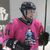# Once() not working in new body::esri:visible column

96
2
05-19-2022 12:46 PMNew Contributor II

In an older (pre- body::esri:visible functionality) survey, I used the once() function in my Relevant expressions to turn fields on as needed, but to retain them if the upstream relevance trigger was changed.  That function seems to no longer work in both the Relevant and body::esri:visible columns.

In both, I'm finding that changing the field that triggers relevance/visibility continually re-evaluates, seeming to ignore the once() function entirely.  Did something change? am I missing something obvious?

1 Solution

Accepted SolutionsbyEsri Regular Contributor

Hello @ByronTsang

One alternative workflow you can use is use a calculate question to store the once calculation, then set the relevancy based on the calculate question.

For example:

 type name label calculation relevant select_one yes_no example2 Example select_one question note example4 Example 4 once(\${example2}) text example3 Example 3 \${example4}='yes'
Thank you,
Zach
2 RepliesbyEsri Regular Contributor

Hello @ByronTsang

One alternative workflow you can use is use a calculate question to store the once calculation, then set the relevancy based on the calculate question.

For example:

 type name label calculation relevant select_one yes_no example2 Example select_one question note example4 Example 4 once(\${example2}) text example3 Example 3 \${example4}='yes'
Thank you,
ZachNew Contributor II

Thanks, Zach - I'll give this a try.